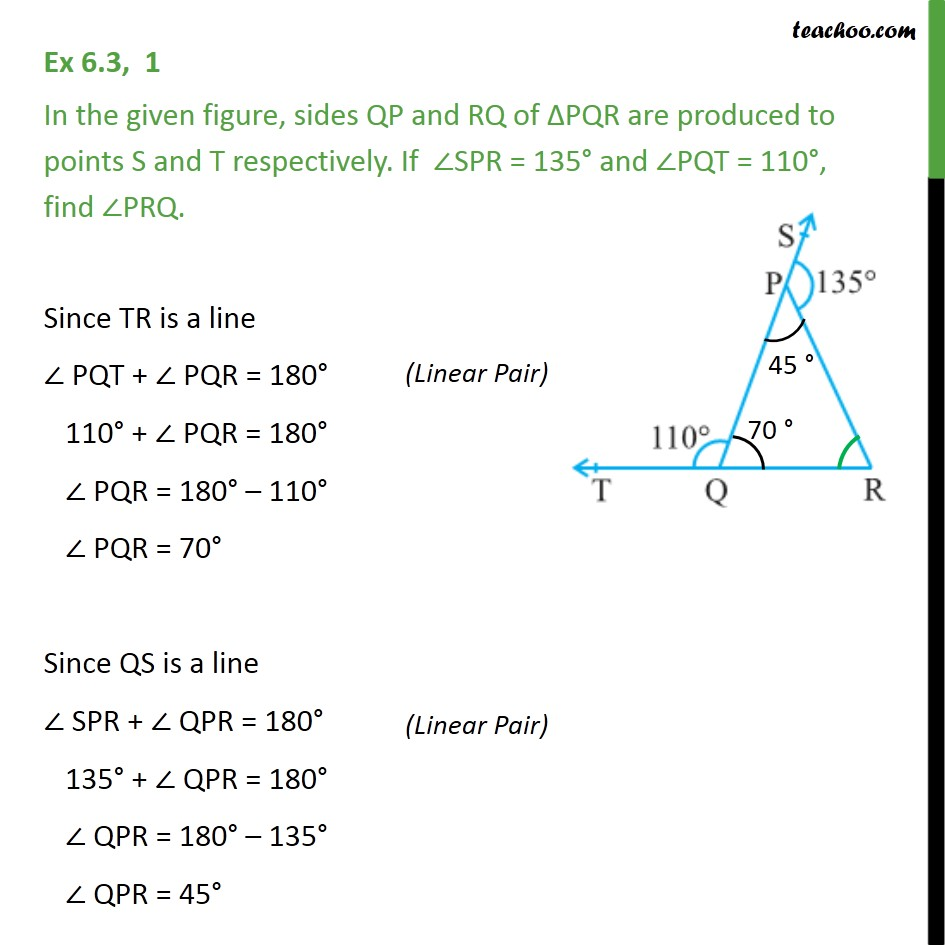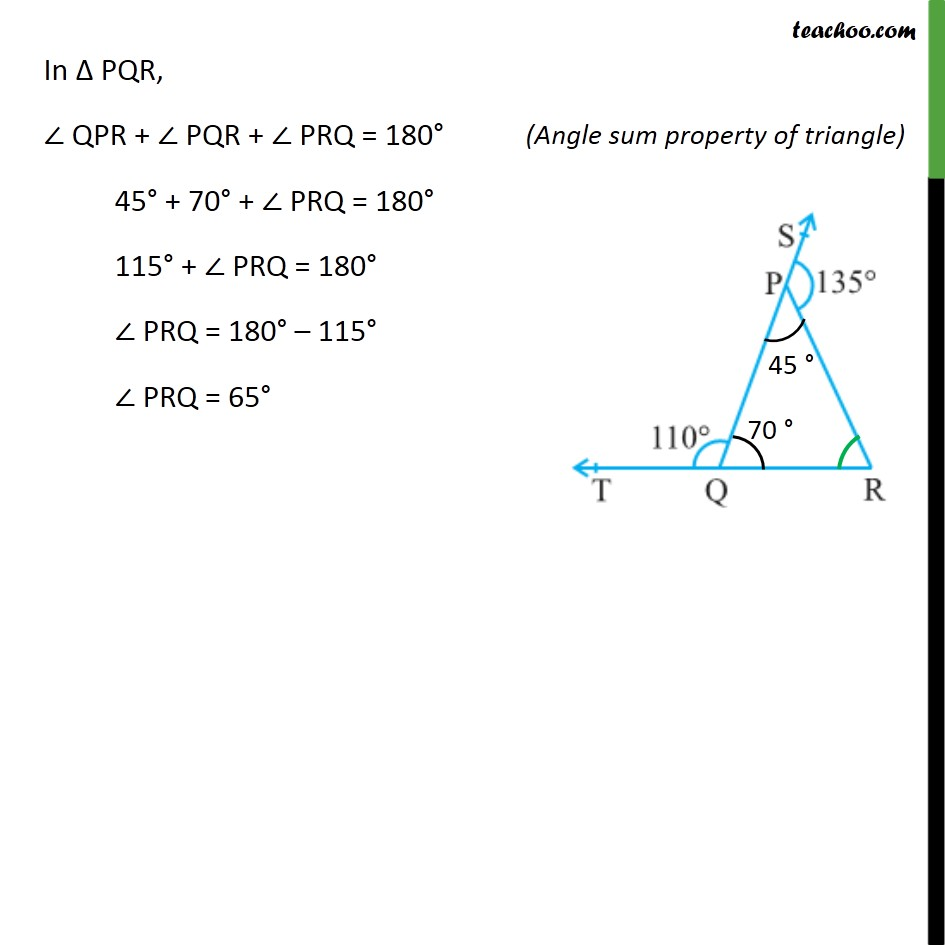1. Chapter 6 Class 9 Lines and Angles (Term 1)
2. Serial order wise
3. Ex 6.3

Transcript

Ex 6.3, 1 In the given figure, sides QP and RQ of ΔPQR are produced to points S and T respectively. If ∠SPR = 135° and ∠PQT = 110°, find ∠PRQ. Since TR is a line ∠ PQT + ∠ PQR = 180° 110° + ∠ PQR = 180° ∠ PQR = 180° – 110° ∠ PQR = 70° Since QS is a line ∠ SPR + ∠ QPR = 180° 135° + ∠ QPR = 180° ∠ QPR = 180° – 135° ∠ QPR = 45° In Δ PQR, ∠ QPR + ∠ PQR + ∠ PRQ = 180° 45° + 70° + ∠ PRQ = 180° 115° + ∠ PRQ = 180° ∠ PRQ = 180° – 115° ∠ PRQ = 65°